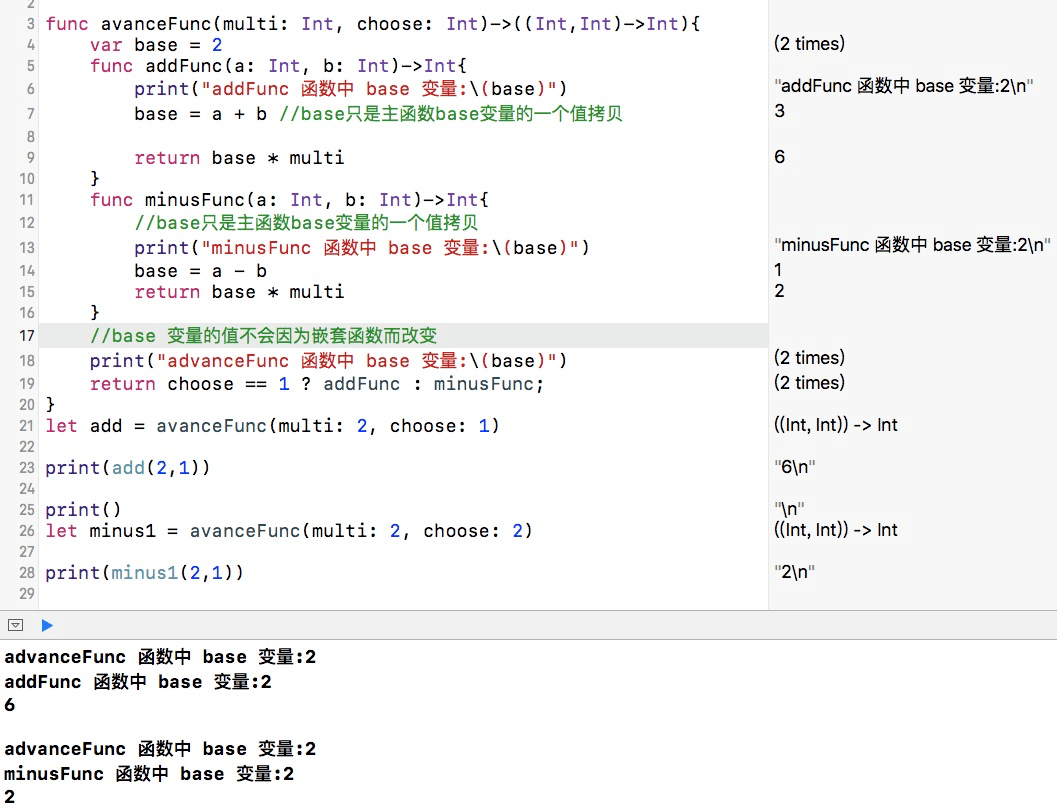# 函数类型

• 在Swift中，每个函数都是一种特定的函数类型，函数类型由函数的参数类型和返回类型组成。使用函数类型就像使用其他类型一样，例如，可以定义一个类型为函数的常量或变量，并且把函数值赋给它。
``````func addTwo( a : Int, b: Int)-> Int{
return a+b
}
var sumTwo: (Int, Int)-> Int = addTwo
sumTwo(1,3)
``````
• 函数类型也可以作为另一个函数的参数进行传递。
``````func printAddTwo(addTwo: (Int,Int)->Int,a: Int, b: Int){
//打印结果:3
}
``````
• 同样的，函数类型可以作为另一个函数的返回值进行返回。
``````func returnAddFunc()->((Int,Int)->Int){
}
``````

# 嵌套函数

``````func returnMinusFunc()->((Int, Int)->Int){
func minusFunc(a: Int, b: Int)->Int{
return a-b
}
return minusFunc
}
let minusTwoInt = returnMinusFunc()
print(minusTwoInt(5,1)) //打印：4
``````

# 示例代码

``````func avanceFunc(multi: Int, choose: Int)->((Int,Int)->Int){
var base = 2
base = a + b //base只是主函数base变量的一个值拷贝

return base * multi
}
func minusFunc(a: Int, b: Int)->Int{
//base只是主函数base变量的一个值拷贝
print("minusFunc 函数中 base 变量:\(base)")
base = a - b
return base * multi
}
//base 变量的值不会因为嵌套函数而改变
return choose == 1 ? addFunc : minusFunc;
}
let add = avanceFunc(multi: 2, choose: 1)

print()
let minus1 = avanceFunc(multi: 2, choose: 2)

print(minus1(2,1))

``````# 示例代码

https://github.com/99ios/23.8